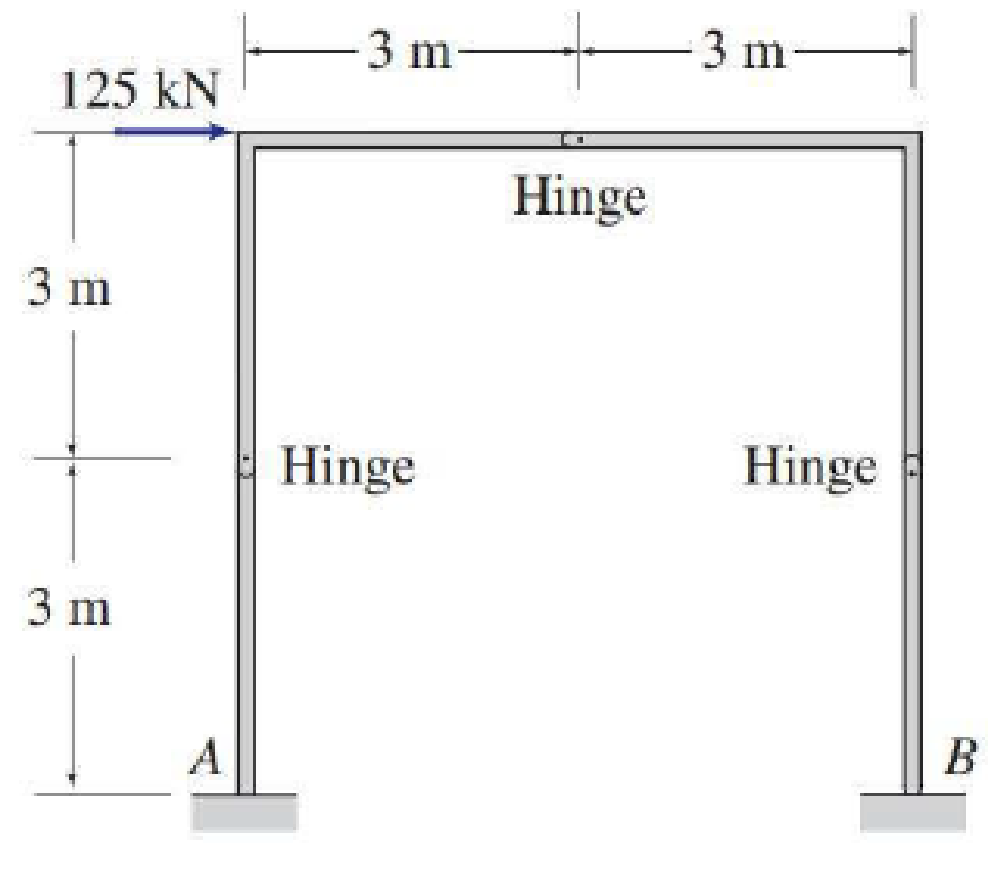# 3.16 through 3.42 Determine the reactions at the supports for the structures shown.

#### Solutions

Chapter
Section
Chapter 3, Problem 41P
Textbook Problem
6 views

## 3.16 through 3.42 Determine the reactions at the supports for the structures shown.To determine

Calculate the support reactions for the given structure.

### Explanation of Solution

Given information:

The structure is given in the Figure.

Apply the sign conventions for calculating reaction forces and moments using the three equations of equilibrium as shown below.

• For summation of forces along x-direction is equal to zero (Fx=0), consider the forces acting towards right side as positive (+) and the forces acting towards left side as negative ().
• For summation of forces along y-direction is equal to zero (Fy=0), consider the upward force as positive (+) and the downward force as negative ().
• For summation of moment about a point is equal to zero (Matapoint=0), consider the clockwise moment as negative and the counter clockwise moment as positive.

Calculation:

Let Ax, Ay, and MA be the horizontal reaction, vertical reaction, and moment at the fixed support A.

Let Bx, By, and MB be the horizontal reaction, vertical reaction, and moment at the fixed support B.

Let Cx and Cy be the horizontal and vertical reaction at the internal hinge C.

Let Dx and Dy be the horizontal and vertical reaction at the internal hinge D.

Sketch the free body diagram of the structure as shown in Figure 1.

Use equilibrium equations:

Find the forces at the internal hinges C and D:

Consider the free body diagram of the portion CED.

Summation of moments about D is equal to 0.

MD=0Cy(6)125(3)=06Cy=375Cy=62.5kN

For the member CE, the summation of moments about E is equal to 0.

MECE=0Cy(3)Cx(3)=0

Substitute 62.5kN for Cy.

62.5(3)Cx(3)=0Cx=62.5kN

For the member CED, the summation of forces along x-direction is equal to 0.

+Fx=0Cx+125Dx=0

Substitute 62.5kN for Cx.

62.5+125Dx=0Dx=62.5kN

For the member CED, the summation of forces along y-direction is equal to 0.

+Fy=0Cy+Dy=0

Substitute 62.5kN for Cy.

62.5+Dy=0Dy=62.5kN

Find the reactions at the support A:

Consider the equilibrium of the portion AC.

Summation of forces along x-direction is equal to 0.

+Fx=0Ax+Cx=0

Substitute 62

### Still sussing out bartleby?

Check out a sample textbook solution.

See a sample solution

#### The Solution to Your Study Problems

Bartleby provides explanations to thousands of textbook problems written by our experts, many with advanced degrees!

Get Started

Find more solutions based on key concepts
Show the steps that you would take to convert your height from feet and inches to meters and centimeters.

Engineering Fundamentals: An Introduction to Engineering (MindTap Course List)

Determine the resultant of the force system shown.

International Edition---engineering Mechanics: Statics, 4th Edition

Differentiate among the following technology user types: home user, small/home office user, mobile user, power ...

Enhanced Discovering Computers 2017 (Shelly Cashman Series) (MindTap Course List)

Identify several specific characteristics of intelligent behavior.

Principles of Information Systems (MindTap Course List)

If your motherboard supports ECC DDR3 memory, can you substitute non-ECC DDR3 memory?

A+ Guide to Hardware (Standalone Book) (MindTap Course List)

What are some skills that entry-level welders need?

Welding: Principles and Applications (MindTap Course List)